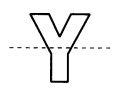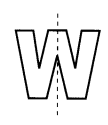# Math in Focus Grade 3 Chapter 18 Practice 3 Answer Key Symmetry

This handy Math in Focus Grade 3 Workbook Answer Key Chapter 18 Practice 3 Symmetry provides detailed solutions for the textbook questions.

## Math in Focus Grade 3 Chapter 18 Practice 3 Answer Key Symmetry

Circle the symmetric figures.

Question 1.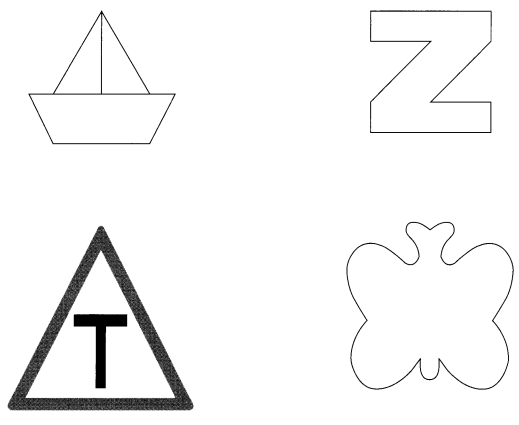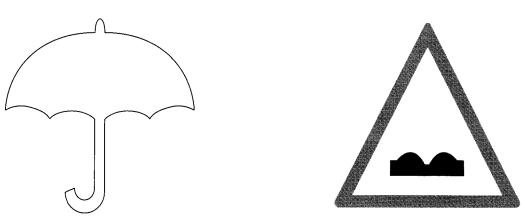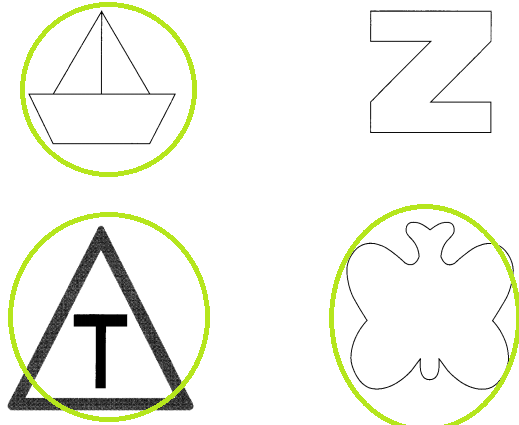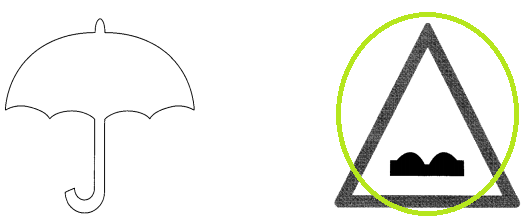Explanation:
The line of symmetry is the axis or imaginary line that passes through the center of the shape or object,
and divides it into identical halves.
The above all figures show symmetry expect the letter N and umbrella.

Decide which of the dotted lines are lines of symmetry.

Question 2.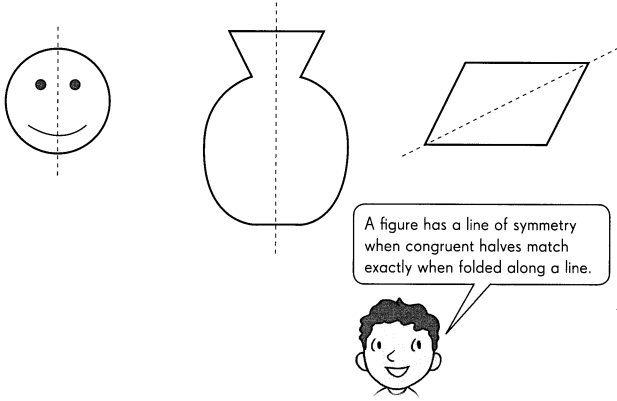All the figures of dotted lines are lines of symmetry.
Explanation:
The line of symmetry is the axis or imaginary line that passes through the center of the shape or object and divides it into identical halves.

Decide whether the line is a line of symmetry. Write yes or no.

Question 3.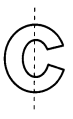Explanation:
The line of symmetry is the axis or imaginary line that passes through the center of the shape or object and divides it into identical halves.
the above figure doesn’t show line of symmetry.

Question 4.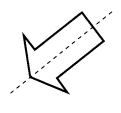Explanation:
The line of symmetry is the axis or imaginary line that passes through the center of the shape or object and divides it into identical halves.
The above figure shows line of symmetry.

Question 5.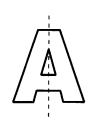Explanation:
The line of symmetry is the axis or imaginary line that passes through the center of the shape or object and divides it into identical halves.
The above figure shows line of symmetry.

Question 6.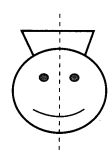Explanation:
The line of symmetry is the axis or imaginary line that passes through the center of the shape or object and divides it into identical halves.
The above figure shows line of symmetry.

Question 7.